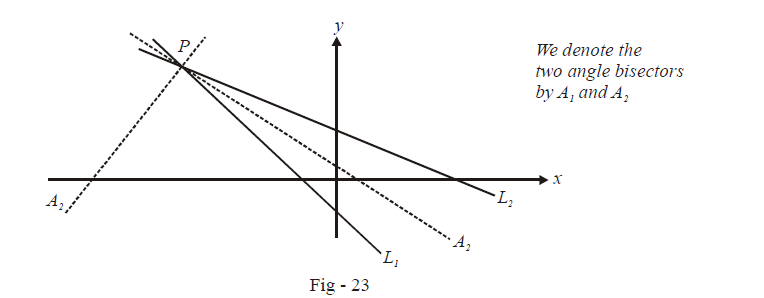# Angle Bisectors of Angles Formed by Intersecting Lines

Go back to  'Straight Lines'

$$\textbf{Art 9 :} \qquad\boxed{\text{Angle Bisectors}}$$

Consider two straight lines L1 and L2 with the equations

\begin{align}{L_1}\,\,\,\,:\,\,\,{a_1}x + {b_1}y + {c_1} = 0\\{L_2}\,\,\,\,:\,\,\,{a_2}x + {b_2}y + {c_2} = 0\end{align}

We intend to find the angle bisector formed at the intersection point P of L1 and L2. Note that there will be two such angle bisectorsTo write down the equations of the two angle bisectors, we first modify the equations of L1 and L2 so that c1 and c2 are say, both negative in sign. This can always be done. Why this is done will soon become clear.

We first write down the equation of A1, the angle bisector of the angle in which the origin lies.

By virtue of being an angle bisector, if any point $$P(x',y')$$ lies on A1, the distance of P from L1 and L2 must be equal. Using the perpendicular distance formula of Art -7, we have

\begin{align}&\Rightarrow\qquad \left| {\frac{{{a_1}x' + {b_1}y' + {c_1}}}{{\sqrt {a_1^{\,\,2} + b_1^{\,\,2}} }}} \right| = \left| {\frac{{{a_2}x' + {b_2}y' + {c_2}}}{{\sqrt {a_2^{\,\,2} + b_2^{\,\,2}} }}} \right|\\&\Rightarrow\qquad\frac{{{a_1}x' + {b_1}y' + {c_1}}}{{\sqrt {a_1^{\,\,2} + b_1^{\,\,2}} }} = \pm \frac{{{a_2}x' + {b_2}y' + {c_2}}}{{\sqrt {a_2^{\,\,2} + b_2^{\,\,2}} }} \qquad \qquad \qquad \qquad \dots \rm{(1)}\end{align}

Which sign should we select, “+” or “–”, for the bisector of the angle containing the origin?

Since P and origin lie on the same side of $${L_1,}\,{a_1}x' + {b_1}y' + {c_1}$$ and c1 must be of the same sign by Art - 6. Similarly, $${a_2}x' + {b_2}y' + {c_2}$$ and c2 must be of the same sign. But since we have already arranged c1 and c2 to be of the same sign (both negative), we must have $$\left( {{a_1}x' + {b_1}y' + {c_1}} \right)$$ and $$\left( {{a_2}x' + {b_2}y' + {c_2}} \right)$$ also of the same sign.

Thus, it follows from (1) that to write the equation of the angle bisector of the angle containing the origin, we must select the “+” sign since $$\left( {{a_1}x' + {b_1}y' + {c_1}} \right)$$ and $$\left( {{a_2}x' + {b_2}y' + {c_2}} \right)$$ are of the same sign. The “–” sign gives the angle bisector of the angle not containing the origin, i.e., the equation of A2.

To summarize, we first arrange the equations of Land L2 so that c1 and c2 are both of the same sign. Subsequently, using the property of any angle bisector, we obtain

\begin{align}&\boxed{{\frac{{{a_1}x + {b_1}y + {c_1}}}{{\sqrt {a_1^{\,\,2} + b_1^{\,\,2}} }} = + \frac{{{a_2}x + {b_2}y + {c_2}}}{{\sqrt {a_2^{\,\,2} + b_2^{\,\,2}} }}}}\qquad \qquad: \qquad \qquad \begin{array}{l}{\textbf{Angle bisector of angle}}\\{\textbf{contaning the origin}}\end{array}\\\\& \qquad \qquad \text {and}\\\\ &\boxed{{\frac{{{a_1}x + {b_1}y + {c_1}}}{{\sqrt {a_1^{\,\,2} + b_1^{\,\,2}} }} = - \frac{{{a_2}x + {b_2}y + {c_2}}}{{\sqrt {a_2^{\,\,2} + b_2^{\,\,2}} }}}}\qquad \qquad: \qquad \qquad \begin{array}{l}{\textbf{Angle bisector of angle not}}\\{\textbf{contaning the origin}}\end{array}\end{align}